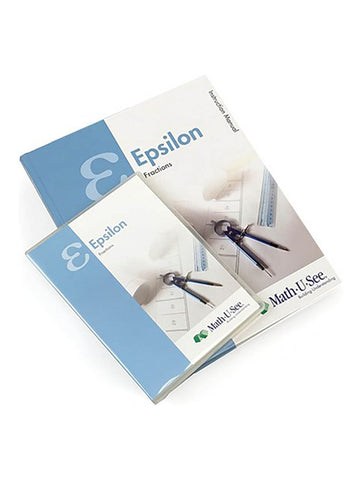# Math-U-See Epsilon Instruction Pack

• \$ 57.00

The Epsilon Instruction Pack contains the Instruction Manual with lesson-by-lesson instructions and full solutions, and the DVD with lesson-by-lesson video instruction.

Now that students have learned basic operations with whole numbers, Epsilon covers these same operations with fractions. Fractions are presented in an intuitive way with visual explanations of equivalent fractions, common denominators, and fractions and numbers larger than 1. Fractions and operations are illustrated using our proprietary Fraction Overlay manipulatives.